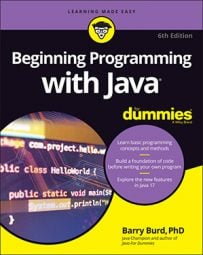##### Beginning Programming with Java For DummiesMost Java programs don’t work correctly the first time you run them, and some programs don’t work without extensive trial and error on your part. This code is a case in point.

To write this code, you need a way to generate three-letter words randomly. This code would give you the desired result:

```anAccount.lastName = " +
(char) (myRandom.nextInt(26) + 'A') +
(char) (myRandom.nextInt(26) + 'a') +
(char) (myRandom.nextInt(26) + 'a');```
Here’s how the code works:
• Each call to the Random.nextInt(26) generates a number from 0 to 25.

• Adding 'A' gives you a number from 65 to 90.

To store a letter 'A', the computer puts the number 65 in its memory. That’s why adding 'A' to 0 gives you 65 and why adding 'A' to 25 gives you 90.

• Applying (char) to a number turns the number into a char value.

To store the letters 'A' through 'Z', the computer puts the numbers 65 through 90 in its memory. So applying (char) to a number from 65 to 90 turns the number into an uppercase letter.

Pause for a brief summary. The expression (char) (myRandom.nextInt(26) + 'A') represents a randomly generated uppercase letter. In a similar way, (char) (myRandom.nextInt(26) + 'a') represents a randomly generated lowercase letter.

Watch out! The next couple of steps can be tricky.

• Java doesn’t allow you to assign a char value to a string variable.

The following statement would lead to a compiler error:

```//Bad statement:
anAccount.lastName = (char) (myRandom.nextInt(26) + 'A');```
• In Java, you can use a plus sign to add a char value to a string. When you do, the result is a string.

So "" + (char) (myRandom.nextInt(26) + 'A') is a string containing one randomly generated uppercase character. And when you add (char) (myRandom.nextInt(26) + 'a') onto the end of that string, you get another string — a string containing two randomly generated characters

Finally, when you add another (char) (myRandom.nextInt(26) + 'a') onto the end of that string, you get a string containing three randomly generated characters. So you can assign that big string to anAccount.lastName. That’s how the statement works.

When you write a program, you have to be very careful with numbers, char values, and strings. This isn’t the kind of programming you do every day of the week, but it is a lesson that you need to be persistent in your Java programming.